GATE  >  Test: Determinacy & Indeterminacy -2

# Test: Determinacy & Indeterminacy -2

Test Description

## 10 Questions MCQ Test GATE Civil Engineering (CE) 2023 Mock Test Series | Test: Determinacy & Indeterminacy -2

Test: Determinacy & Indeterminacy -2 for GATE 2023 is part of GATE Civil Engineering (CE) 2023 Mock Test Series preparation. The Test: Determinacy & Indeterminacy -2 questions and answers have been prepared according to the GATE exam syllabus.The Test: Determinacy & Indeterminacy -2 MCQs are made for GATE 2023 Exam. Find important definitions, questions, notes, meanings, examples, exercises, MCQs and online tests for Test: Determinacy & Indeterminacy -2 below.
Solutions of Test: Determinacy & Indeterminacy -2 questions in English are available as part of our GATE Civil Engineering (CE) 2023 Mock Test Series for GATE & Test: Determinacy & Indeterminacy -2 solutions in Hindi for GATE Civil Engineering (CE) 2023 Mock Test Series course. Download more important topics, notes, lectures and mock test series for GATE Exam by signing up for free. Attempt Test: Determinacy & Indeterminacy -2 | 10 questions in 30 minutes | Mock test for GATE preparation | Free important questions MCQ to study GATE Civil Engineering (CE) 2023 Mock Test Series for GATE Exam | Download free PDF with solutions
 1 Crore+ students have signed up on EduRev. Have you?
Test: Determinacy & Indeterminacy -2 - Question 1

### If 4 reactions are acting on a beam, then the system is:-

Detailed Solution for Test: Determinacy & Indeterminacy -2 - Question 1

4 reactions mean that the system is definitely indeterminate. But stability would depend upon the nature of forces acting on the planar structure.

Test: Determinacy & Indeterminacy -2 - Question 2

### A prismatic beam is shown in the figure given below.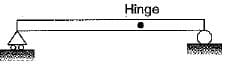​Consider the following statements: 1. The structure is unstable. 2. The bending moment is zero at supports and internal hinge. 3. It is a mechanism, 4. It is statically indeterminate. Which of these statements are correct?

Detailed Solution for Test: Determinacy & Indeterminacy -2 - Question 2

The degree of indeterminacy = 3 - 1 - 1 = -1 V So structure is deficient.and unstable. It is a mechanism,

Test: Determinacy & Indeterminacy -2 - Question 3

### The rigid-jointed frame shown in the figure is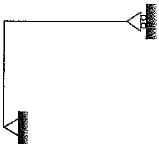Test: Determinacy & Indeterminacy -2 - Question 4

What is the degree of static indeterminacy of the plane structure as shown in the figure below?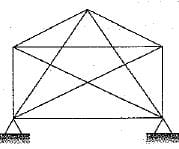Detailed Solution for Test: Determinacy & Indeterminacy -2 - Question 4

For plane truss degree of indeterminacy,
Ds = m + re - 2j
re = 4; m= 10; j = 5
Ds = 4 + 10 - 2 x 5 = 4

Test: Determinacy & Indeterminacy -2 - Question 5

Consider the following statements:
1. The displacement method is more useful when degree of kinematic indeterminacy is greater than the degree of static indeterminacy,
2. The displacement method is more useful when degree of kinematic indeterminacy is less than degree of static indeterminacy.
3. The force method is more useful when degree of static indeterminacy is greater than the degree of kinematic indeterminacy
4. The force method is more useful when degree of static indeterminacy is less than the degree of kinematic indeterminacy

Which of these statements is correct?

Detailed Solution for Test: Determinacy & Indeterminacy -2 - Question 5

Force method is useful when,
Ds < Dk
Displacement method is useful when,
Dk < Ds

Test: Determinacy & Indeterminacy -2 - Question 6

Which one of the following structures is statically determinate and stable?

Detailed Solution for Test: Determinacy & Indeterminacy -2 - Question 6

A structure will be statically determinate if the external reactions can be determined from force- equilibrium equations. A structure is stable when the whole or part of the structure is prevented from large displacements on account of loading.
The structure in figure (b) is stable but statically indeterminate to the second degree.
The structure shown in figure (c) has both reaction components coinciding with each other, so the moment equilibrium condition wilt never be satisfied and the structure will not be under equilibrium. In figure'(a), the structure is stable and there are three reaction components which can be determined by two force equilibrium conditions and one moment equilibrium condition.

Test: Determinacy & Indeterminacy -2 - Question 7

How is a truss, which undergoes rigid body translation for an arbitrary load, classified as?

Test: Determinacy & Indeterminacy -2 - Question 8

The degree of static indeterminacy of the rigid frame having two internal hinges as shown in the figure below is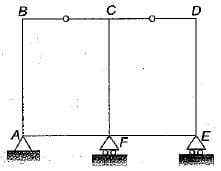Detailed Solution for Test: Determinacy & Indeterminacy -2 - Question 8

Ds=Dse+Dsi
Dss= rE- 3
= 4 - 3 = 1
Dsi = 3C - r= 3C - ∑(m1- 1)
= 3 x 2 -(2-1) +(2-1)
= 6 - 2 = 4
∴ Ds = Dss + Dsi = 5

Test: Determinacy & Indeterminacy -2 - Question 9

The pin-jointed frame shown in the figure is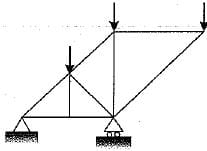Detailed Solution for Test: Determinacy & Indeterminacy -2 - Question 9

Degree of indeterminacy,
n = (m + rE) - 2j
= (9 + 3 ) - 2 x 6 = 0
Since the degree of indeterminacy is zero and the frame is stable so it is a perfect frame.

Test: Determinacy & Indeterminacy -2 - Question 10

What is the total degree of indeterminacy both internal and external of the plane frame shown below?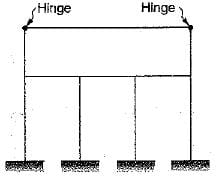Detailed Solution for Test: Determinacy & Indeterminacy -2 - Question 10

Ds = 3m + re- rr 3(j + j)
Number of members m=10
Number of external reactions re= 12
Number of interna! reaction components released
(rr) = 2
Number of rigid joints (j) = 8
Number of joints at which releases are located (j') = 2
∴ Ds = 3 x 10 + 12 - 2 - 3 x ( 8 + 2) = 10

## GATE Civil Engineering (CE) 2023 Mock Test Series

26 docs|292 tests
 Use Code STAYHOME200 and get INR 200 additional OFF Use Coupon Code
Information about Test: Determinacy & Indeterminacy -2 Page
In this test you can find the Exam questions for Test: Determinacy & Indeterminacy -2 solved & explained in the simplest way possible. Besides giving Questions and answers for Test: Determinacy & Indeterminacy -2, EduRev gives you an ample number of Online tests for practice

## GATE Civil Engineering (CE) 2023 Mock Test Series

26 docs|292 tests